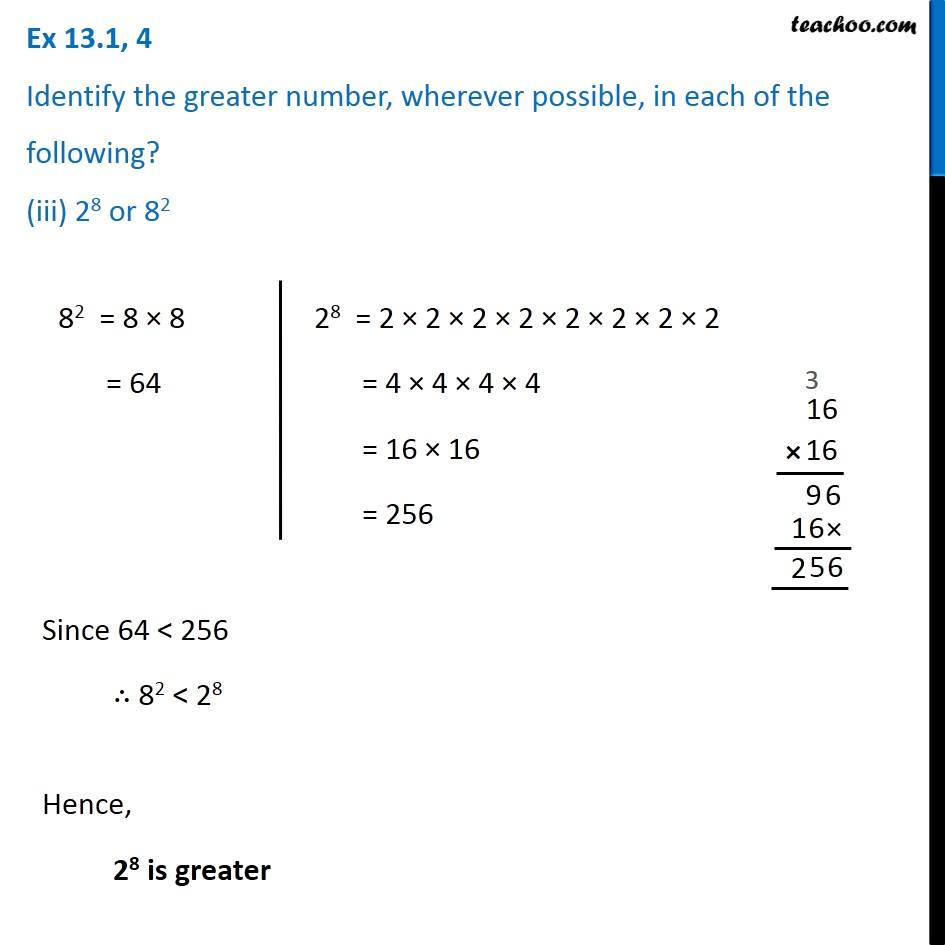Ex 13.1

Chapter 13 Class 7 Exponents and Powers
Serial order wiseIntroducing your new favourite teacher - Teachoo Black, at only ₹83 per month

### Transcript

Ex 13.1, 4 Identify the greater number, wherever possible, in each of the following? (iii) 28 or 82 82 = 8 × 8 = 64 28 = 2 × 2 × 2 × 2 × 2 × 2 × 2 × 2 = 4 × 4 × 4 × 4 = 16 × 16 = 256 Since 64 < 256 ∴ 82 < 28 Hence, 28 is greater